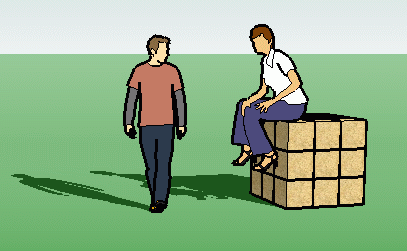SEARCH HOMEMath Central Quandaries & QueriesQuestion from George: How many cubic feet in a cubic yard?George,A cubic yard is a yard cubed. So

(1 yard )3 = 13 yard3 = 1 yard3 = 1 cubic yard .

Since 1 yard = 3 feet, we have

1 cubic yard = (1 yard)3 = (3 feet)3 = 33 feet3 = 27 cubic feet.

Simply put, when dealing with square or cubic units, you square or cube the appropriate conversion factor.

Cheers,
Stephen La Rocque.Math Central is supported by the University of Regina and The Pacific Institute for the Mathematical Sciences.